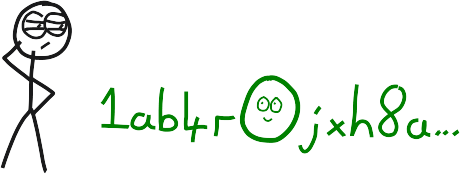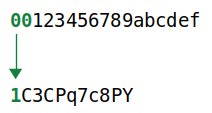Base58
• Hash256
• Hash160
• Reverse Bytes

# Base58 A set of characters that's easy to share.

``````  1 2 3 4 5 6 7 8 9
A B C D E F G H   J K L M N   P Q R S T U V W X Y Z
a b c d e f g h i j k   m n o p q r s t u v w x y z``````

Base58 is a set of characters you can use to represent big numbers in a shorter and more user-friendly format.

## What does base58 mean?

The "base" refers to the number of characters you use to represent a number.

Base Characters
2 (binary) `01`
10 `0123456789`
16 (hexadecimal) `0123456789abcdef`
58 ` 123456789ABCDEFGH JKLMN PQRSTUVWXYZabcdefghijk mnopqrstuvwxyz`

So in everyday life, we are used to working with base10 numbers (using the digits `0-9`).

But if you're a computer, it's easy enough to use extra characters to represent numbers:

``````base10(9999) = 9999
base16(9999) = 270f
base58(9999) = 3yQ``````

All of these "numbers" have the same value -- they just use different sets of characters (bases) to represent it.

The more characters you have in your base, the less of them you will need to use to represent big numbers. So the bigger your base, the shorter your "number" can be.

## Why base58?

Because 58 is the number of characters you are left with when you use all the characters in the alphanumeric alphabet (62), but remove all the easily mistakable characters like `0`, `O`, `l` and `I`.

``````alphanumeric = 0123456789ABCDEFGHIJKLMNOPQRSTUVWXYZabcdefghijklmnopqrstuvwxyz
base58       =  123456789ABCDEFGH JKLMN PQRSTUVWXYZabcdefghijk mnopqrstuvwxyz``````

So base58 has two advantages:

1. It gives you a large set of characters, so you can represent large numbers in a shorter format.
2. It leaves out awkward characters, to save you from making mistakes when transcribing.A pesky O/0

## How to convert a number to base58.

To convert an integer (base10) to base58, you use the modulus1 function to work out which characters you need to represent the original number.

Basically, you keep dividing your number by 58, take the remainder at each step of the way to get the index for the next character, and keep going until there are no remainders left.

``````base10 = 123456789

123456789 % 58 = 19
2128565 % 58 = 23
36699 % 58 = 43
632 % 58 = 52
10 % 58 = 10

base58 = 
base58 = BukQL``````Base58 (simple)

``````# A simple function that converts an _integer_ to base58:

def base58(i)

@characters = %w[
1 2 3 4 5 6 7 8 9
A B C D E F G H   J K L M N   P Q R S T U V W X Y Z
a b c d e f g h i j k   m n o p q r s t u v w x y z
]

# create an empty string (in preparation to hold the new characters)
buffer = ''

# keep finding the remainder until our starting number hits zero
while i > 0
# find the remainder after dividing by 58 (% = modulus)
remainder = i % 58

# add the base58 character to the start of the string
buffer = @characters[remainder] + buffer

# divide our integer by 58, and repeat...
i = i / 58
end

return buffer

end

puts base58(9999)``````

## Base58 in Bitcoin

Base58 is used when you want to convert commonly used things in bitcoin in to an easier-to-share format. For example:

1. WIF Private Keys
• A private key is like a "master password", and you can use it when you want to import bitcoins in to a new wallet. For this occassion, there is such a thing as a WIF Private Key, which is basically a private key in base58.
• A public key is the "public" counterpart to a private key, and you use them when you want to send bitcoins to someone, so it's expected that you're going to type one out from time to time. However, public keys are quite lengthy, so we convert them to Addresses instead, which makes use of base58 in the final step of the conversion.

### Leading Zeros (`0x00...`)

Bitcoin Base58 also converts every byte of zeros (0x00) at the start of a number to a `1`.You see, putting zeros at the start of a number does not increase the size of the number (e.g. `0x12` is the same as `0x0012`), so any zeros at the start of a number would be "lost" in a conversion to base58.

Therefore, to ensure that leading zeros appear in the result, the bitcoin base58 encoding includes an additional step to convert all leading `0x00`'s to `1`'s

Bitcoin Base58

``````module Base58

@chars = %w[
1 2 3 4 5 6 7 8 9
A B C D E F G H   J K L M N   P Q R S T U V W X Y Z
a b c d e f g h i j k   m n o p q r s t u v w x y z
]
@base = @chars.length

def self.encode(hex)
i = hex.to_i(16)
buffer = String.new

while i > 0
remainder = i % @base
i = i / @base
buffer = @chars[remainder] + buffer
end

# add '1's to the start based on number of leading bytes of zeros
leading_zero_bytes = (hex.match(/^(+)/) ? \$1 : '').size / 2

end

end

hex = '0093ce48570b55c42c2af816aeaba06cfee1224faebb6127fe'
puts Base58.encode(hex)``````

### Modulus (%)

The modulus (`%`) function is a sister of the divide (`/`) function. It gives you the remainder of a division:

``````7 % 6 = 1
7 % 5 = 2
7 % 4 = 3
7 % 3 = 1``````

### Source

• `src/base58.cpp`

### Resources

``` Load Time: 0.07678318```